The OpenFOAM FoundationSTARCDCoordinateRotation Class Reference

A coordinateRotation defined by the STAR-CD convention. More...

Inheritance diagram for STARCDCoordinateRotation: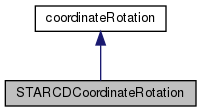[legend]
Collaboration diagram for STARCDCoordinateRotation:[legend]

## Public Member Functions

TypeName ("STARCDRotation")
Runtime type information. More...

STARCDCoordinateRotation ()
Construct null. More...

STARCDCoordinateRotation (const vector &rotZrotXrotY, const bool inDegrees=true)
Construct from rotation vector. More...

STARCDCoordinateRotation (const scalar rotZ, const scalar rotX, const scalar rotY, const bool inDegrees=true)
Construct from components of rotation vector. More...

STARCDCoordinateRotation (const dictionary &)
Construct from dictionary. More...

STARCDCoordinateRotation (const dictionary &, const objectRegistry &)
Construct from dictionary and mesh. More...

virtual void clear ()
Reset rotation to an identity rotation. More...

virtual void updateCells (const polyMesh &, const labelList &)
Update the rotation for a list of cells. More...

virtual const tensorR () const
Return local-to-global transformation tensor. More...

virtual const tensorRtr () const
Return global-to-local transformation tensor. More...

virtual const vector e1 () const
Return local Cartesian x-axis in global coordinates. More...

virtual const vector e2 () const
Return local Cartesian y-axis in global coordinates. More...

virtual const vector e3 () const
Return local Cartesian z-axis in global coordinates. More...

virtual const tensorFieldTr () const
Return transformation tensor field. More...

virtual tmp< vectorFieldtransform (const vectorField &st) const
Transform vectorField using transformation tensor field. More...

virtual vector transform (const vector &st) const
Transform vector using transformation tensor. More...

virtual tmp< vectorFieldinvTransform (const vectorField &st) const
Inverse transform vectorField using transformation tensor field. More...

virtual vector invTransform (const vector &st) const
Inverse transform vector using transformation tensor. More...

virtual tmp< tensorFieldtransformTensor (const tensorField &st) const
Transform tensor field using transformation tensorField. More...

virtual tensor transformTensor (const tensor &st) const
Transform tensor using transformation tensorField. More...

virtual tmp< tensorFieldtransformTensor (const tensorField &st, const labelList &cellMap) const
Transform tensor sub-field using transformation tensorField. More...

virtual tmp< symmTensorFieldtransformVector (const vectorField &st) const
Transform vectorField using transformation tensorField and return. More...

virtual symmTensor transformVector (const vector &st) const
Transform vector using transformation tensor and return. More...

virtual void write (Ostream &) const
Write. More...Public Member Functions inherited from coordinateRotation
TypeName ("coordinateRotation")
Runtime type information. More...

declareRunTimeSelectionTable (autoPtr, coordinateRotation, objectRegistry,(const dictionary &dict, const objectRegistry &obr),(dict, obr))

declareRunTimeSelectionTable (autoPtr, coordinateRotation, dictionary,(const dictionary &dict),(dict))

virtual ~coordinateRotation ()
Destructor. More...

virtual bool uniform () const
Return true if the rotation tensor is uniform. More...Static Public Member Functions inherited from coordinateRotation
static autoPtr< coordinateRotationNew (const dictionary &dict, const objectRegistry &obr)
Select constructed from dictionary and objectRegistry. More...

static autoPtr< coordinateRotationNew (const dictionary &dict)
Select constructed from dictionary. More...Protected Member Functions inherited from coordinateRotation
symmTensor transformPrincipal (const tensor &, const vector &) const
Transform principal. More...

## Detailed Description

A coordinateRotation defined by the STAR-CD convention.

The 3 rotations are defined in the STAR-CD convention (around Z, around X' and around Y''). The order of the parameter arguments matches this rotation order.

• the rotation angles are in degrees, unless otherwise explicitly specified:
```coordinateRotation
{
type        STARCDRotation;
degrees     false;
rotation    (0 0 3.141592654);
}
```
Source files

Definition at line 63 of file STARCDCoordinateRotation.H.

## ◆ STARCDCoordinateRotation() [1/5]

 STARCDCoordinateRotation ( )

Construct null.

Definition at line 195 of file STARCDCoordinateRotation.C.

Referenced by STARCDCoordinateRotation::STARCDCoordinateRotation().

Here is the caller graph for this function: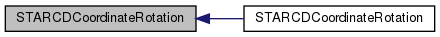## ◆ STARCDCoordinateRotation() [2/5]

 STARCDCoordinateRotation ( const vector & rotZrotXrotY, const bool inDegrees = `true` )

Construct from rotation vector.

Definition at line 203 of file STARCDCoordinateRotation.C.

Here is the call graph for this function: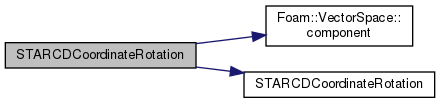## ◆ STARCDCoordinateRotation() [3/5]

 STARCDCoordinateRotation ( const scalar rotZ, const scalar rotX, const scalar rotY, const bool inDegrees = `true` )

Construct from components of rotation vector.

Definition at line 222 of file STARCDCoordinateRotation.C.

Here is the call graph for this function: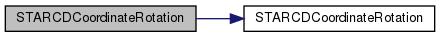## ◆ STARCDCoordinateRotation() [4/5]

 STARCDCoordinateRotation ( const dictionary & dict )

Construct from dictionary.

Definition at line 237 of file STARCDCoordinateRotation.C.

Here is the call graph for this function: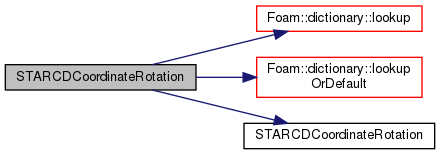## ◆ STARCDCoordinateRotation() [5/5]

 STARCDCoordinateRotation ( const dictionary & dict, const objectRegistry & )

Construct from dictionary and mesh.

Definition at line 257 of file STARCDCoordinateRotation.C.

Here is the call graph for this function: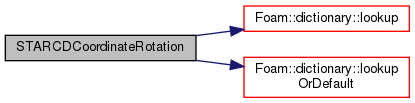## ◆ TypeName()

 TypeName ( "STARCDRotation" )

Runtime type information.

## ◆ clear()

 virtual void clear ( )
inlinevirtual

Reset rotation to an identity rotation.

Implements coordinateRotation.

Definition at line 125 of file STARCDCoordinateRotation.H.

References SphericalTensor< Cmpt >::I.

## ◆ updateCells()

 virtual void updateCells ( const polyMesh & , const labelList & )
inlinevirtual

Update the rotation for a list of cells.

Implements coordinateRotation.

Definition at line 132 of file STARCDCoordinateRotation.H.

## ◆ R()

 virtual const tensor& R ( ) const
inlinevirtual

Return local-to-global transformation tensor.

Implements coordinateRotation.

Definition at line 136 of file STARCDCoordinateRotation.H.

## ◆ Rtr()

 virtual const tensor& Rtr ( ) const
inlinevirtual

Return global-to-local transformation tensor.

Implements coordinateRotation.

Definition at line 142 of file STARCDCoordinateRotation.H.

## ◆ e1()

 virtual const vector e1 ( ) const
inlinevirtual

Return local Cartesian x-axis in global coordinates.

Implements coordinateRotation.

Definition at line 148 of file STARCDCoordinateRotation.H.

References Tensor< Cmpt >::x().

Referenced by STARCDCoordinateRotation::write().

Here is the call graph for this function: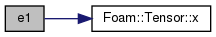Here is the caller graph for this function: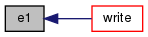## ◆ e2()

 virtual const vector e2 ( ) const
inlinevirtual

Return local Cartesian y-axis in global coordinates.

Implements coordinateRotation.

Definition at line 154 of file STARCDCoordinateRotation.H.

References Tensor< Cmpt >::y().

Referenced by STARCDCoordinateRotation::write().

Here is the call graph for this function: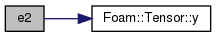Here is the caller graph for this function: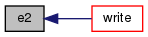## ◆ e3()

 virtual const vector e3 ( ) const
inlinevirtual

Return local Cartesian z-axis in global coordinates.

Implements coordinateRotation.

Definition at line 160 of file STARCDCoordinateRotation.H.

Referenced by STARCDCoordinateRotation::write().

Here is the call graph for this function: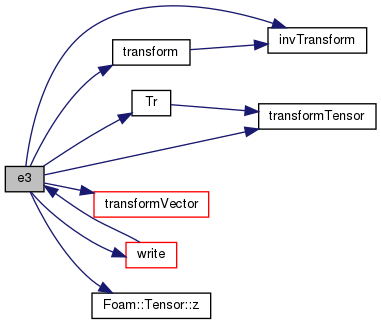Here is the caller graph for this function: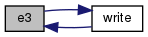## ◆ Tr()

 const Foam::tensorField & Tr ( ) const
virtual

Return transformation tensor field.

Implements coordinateRotation.

Definition at line 88 of file STARCDCoordinateRotation.C.

References NotImplemented, and STARCDCoordinateRotation::transformTensor().

Referenced by STARCDCoordinateRotation::e3().

Here is the call graph for this function: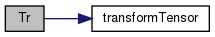Here is the caller graph for this function: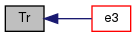## ◆ transform() [1/2]

 Foam::tmp< Foam::vectorField > transform ( const vectorField & st ) const
virtual

Transform vectorField using transformation tensor field.

Implements coordinateRotation.

Definition at line 69 of file STARCDCoordinateRotation.C.

References STARCDCoordinateRotation::invTransform(), and NotImplemented.

Referenced by STARCDCoordinateRotation::e3(), and STARCDCoordinateRotation::invTransform().

Here is the call graph for this function: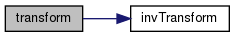Here is the caller graph for this function: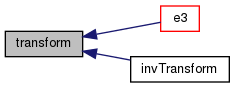## ◆ transform() [2/2]

 Foam::vector transform ( const vector & st ) const
virtual

Transform vector using transformation tensor.

Implements coordinateRotation.

Definition at line 53 of file STARCDCoordinateRotation.C.

References STARCDCoordinateRotation::invTransform().

Here is the call graph for this function:## ◆ invTransform() [1/2]

 Foam::tmp< Foam::vectorField > invTransform ( const vectorField & st ) const
virtual

Inverse transform vectorField using transformation tensor field.

Implements coordinateRotation.

Definition at line 79 of file STARCDCoordinateRotation.C.

References NotImplemented.

Referenced by STARCDCoordinateRotation::e3(), and STARCDCoordinateRotation::transform().

Here is the caller graph for this function: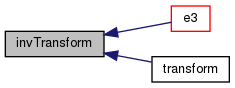## ◆ invTransform() [2/2]

 Foam::vector invTransform ( const vector & st ) const
virtual

Inverse transform vector using transformation tensor.

Implements coordinateRotation.

Definition at line 60 of file STARCDCoordinateRotation.C.

References STARCDCoordinateRotation::transform().

Here is the call graph for this function: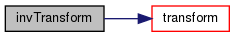## ◆ transformTensor() [1/3]

 Foam::tmp< Foam::tensorField > transformTensor ( const tensorField & st ) const
virtual

Transform tensor field using transformation tensorField.

Implements coordinateRotation.

Definition at line 96 of file STARCDCoordinateRotation.C.

References NotImplemented.

Here is the caller graph for this function: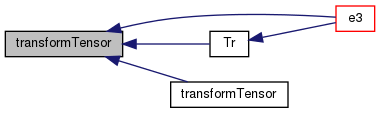## ◆ transformTensor() [2/3]

 Foam::tensor transformTensor ( const tensor & st ) const
virtual

Transform tensor using transformation tensorField.

Implements coordinateRotation.

Definition at line 106 of file STARCDCoordinateRotation.C.

References STARCDCoordinateRotation::transformTensor().

Here is the call graph for this function: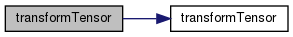## ◆ transformTensor() [3/3]

 Foam::tmp< Foam::tensorField > transformTensor ( const tensorField & st, const labelList & cellMap ) const
virtual

Transform tensor sub-field using transformation tensorField.

Implements coordinateRotation.

Definition at line 115 of file STARCDCoordinateRotation.C.

References NotImplemented, and STARCDCoordinateRotation::transformVector().

Here is the call graph for this function: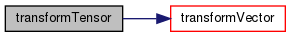## ◆ transformVector() [1/2]

 Foam::tmp< Foam::symmTensorField > transformVector ( const vectorField & st ) const
virtual

Transform vectorField using transformation tensorField and return.

symmetrical tensorField

Implements coordinateRotation.

Definition at line 127 of file STARCDCoordinateRotation.C.

References fld(), forAll, tmp< T >::ref(), and List< T >::size().

Referenced by STARCDCoordinateRotation::e3(), and STARCDCoordinateRotation::transformTensor().

Here is the call graph for this function: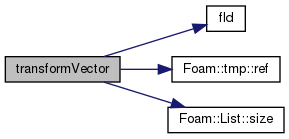Here is the caller graph for this function: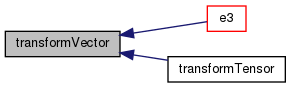## ◆ transformVector() [2/2]

 Foam::symmTensor transformVector ( const vector & st ) const
virtual

Transform vector using transformation tensor and return.

symmetrical tensor

Implements coordinateRotation.

Definition at line 143 of file STARCDCoordinateRotation.C.

References Foam::cos(), Foam::constant::mathematical::pi(), Foam::sin(), x, and y.

Here is the call graph for this function: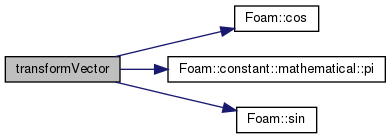## ◆ write()

 void write ( Ostream & os ) const
virtual

Write.

Implements coordinateRotation.

Definition at line 274 of file STARCDCoordinateRotation.C.

Referenced by STARCDCoordinateRotation::e3().

Here is the call graph for this function: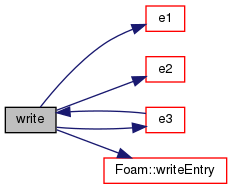Here is the caller graph for this function: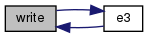The documentation for this class was generated from the following files: